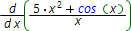# Online derivative calculator

Derivative of a function is the limit of the infinitesimal change in the function with respect to infinitesimal change in the variable, provided that the last tends to zero:To find derivative of some function you'd apply basic differentiation rules or use our online step by step derivative calculator.

It capable to calculate second, third and other higher order derivatives. You can also choose differentiation variable and calculate partial derivatives in case of multivariable functions.

## Choose expression input type:

Expression input type: